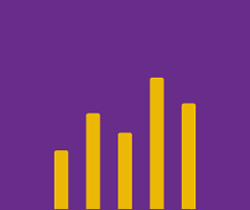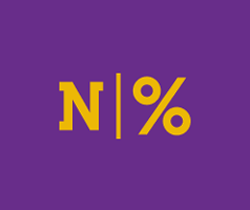# Tutorials: Learn How to Use the Tuva Tools

#### Complete these short interactive tutorials using authentic datasets to learn all the different features of the Tuva data, graphing, and statistical tools.## Tutorials: Creating Graphs & Charts on Tuva

In these short tutorials, students will learn how to create different graphs and charts on Tuva.

12 Lessons 1:41 Hours## Tutorials: Graphing Functions & Creating Models on Tuva

In these short tutorials, students will learn how to graph functions and create linear or nonlinear models within real-world contexts.

5 Lessons 1:10 Hours## Tutorials: Calculating Numerical Measures & Summary Statistics on Tuva

In these short tutorials, students will learn how to use the Count & Percent features on Tuva. They will also learn how to use summary statistics features including visualizing the mean, median, standard deviation, variance, and interquartile range.

3 Lessons 1:10 Hours## Tutorials: Filtering and Other Data Moves on Tuva

In these short tutorials, students will learn how to perform a variety of data moves to filter data, convert a numerical attribute to a categorical attribute, and drag categories on the axis to to group them according to a pattern or by a common trait.

6 Lessons 0:50 Hours## Tutorials: Random Sampling on Tuva

In these tutorials, students will learn how to generate random samples, calculate and visualize sample statistics, and compare sample statistics to population parameters on Tuva.

5 Lessons 1:00 Hours## Tutorials: Using Reference Lines and Dividers on Tuva

In these short tutorials, students will learn how to use reference lines and dividers on Tuva.

1 Lessons 0:10 Hours